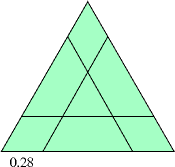# A Pre-Sliced Triangle

Take an equilateral triangle with unit base, oriented so that base is horizontal. Start at the bottom left vertex and go out 0.28 units to the right along the base. Call this point X. From X draw a line parallel to the side not containing the low-left vertex we started at. Then make two additional lines parallel to the other sides of the triangle, so all offsets are the same as for the first parallel line we drew.

Given such a pre-sliced equilateral triangle, are you able to make finitely many additional slices so that the ultimate pieces are all triangles?
--Jim Propp (MIT)Note: A "slice" is a doubly infinite line (and so must pass completely through the triangle).

This problem appeared in the Unsolved Problems section of the latest Amer. Math. Monthly (103 (1996) 342-343, eds: R. Guy & R. Nowakowski). No, the specific case is not unsolved. But the following general problem is:

Given a pre-sliced convex polygon, is it always possible to add finitely many slices so that all the resulting pieces are triangles?
The sketch is rough. More precision: Take any equilateral triangle. Place a small equilateral triangle with same center and in the same orientation as the large triangle. Then extend all sides of the small triangle until they reach the big triangle. The important point is that the interior triangle is to be "small" (the horizontal side is about 0.24 above the base (which has unit length).

If you want more precision, you will have to read or run the Mathematica code that follows. But it is worth it! This is a fun problem!

```Show[Graphics[{AbsolutePointSize,
Line[{{0, 0}, {1, 0}, {0.5, 0.866025}, {0, 0}}],
Line[{{.72, 0}, {.36, 0.623538}}],
Line[{{0.14, 0.242487}, {.86, 0.242487}}],
Line[{{0.28, 0}, {.64, 0.623538} }]  }],
AspectRatio -> Automatic];
```
or just observe that the triangle has vertices at {0, 0}, {1, 0}, and {0.5, 0.866025}, and the three slices are the lines
from {.72, 0} to {.36, 0.623538},
from {0.14, 0.242487} to {0.86, 0.242487}, and
from {0.28, 0} to {.64, 0.623538}.
Even a rough sketch will suffice.
It turns out that the particular case asked, a = 0.28, is not that difficult. Can anyone out there figure out a method that will work for all values of a between 0.29 and 1/3 (1/3 is an upper limit on the diagram)? As far as I can tell, this is an open question, though there are solutions for particular values of a in this range.

Actually, it is fairly simple if a is rational (thanks to John Guilford for pointing this out). So what I really want is a solution in a single irrational case when the central triangle is small (a > 0.293).